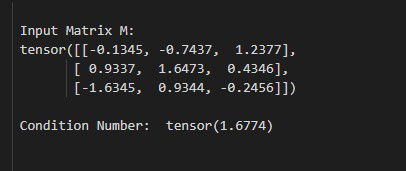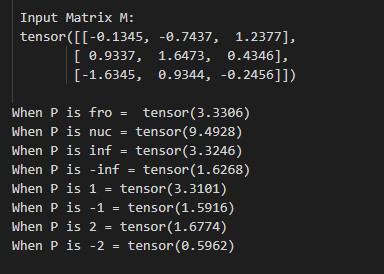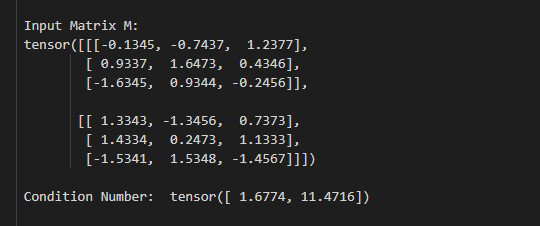GFG App
Open AppBrowser
Continue

# Python PyTorch – torch.linalg.cond() Function

In this article, we are going to discuss how to compute the condition number of a matrix in PyTorch. we can get the condition number of a matrix by using torch.linalg.cond() method.

## torch.linalg.cond() method

This method is used to compute the condition number of a matrix with respect to a matrix norm. This method accepts a matrix and batch of matrices as input. It will return a tensor with a computed condition number. It supports input of double, float, cfloat, and cdouble data types. before moving further let’s see the syntax of this method.

Syntax: torch.linalg.cond(M, P=None)

Parameters:

• M (Tensor): It’s a matrix or a batch of matrices.
• P (int, inf, -inf, ‘fro’, ‘nuc’, optional): It’s defines the matrix norm that is computed. The default value of P 2-norm.

Returns: It will return a tensor with a computed condition number.

### Example 1

In this example, we defined a tensor using torch.tensor, and we will compute the condition number of a matrix with the help of torch.linalg.cond method.

## Python3

 `# import the required library ` `import` `torch ` ` `  `# define a tensor (matrix) ` `M ``=` `torch.tensor([[``-``0.1345``, ``-``0.7437``, ``1.2377``], ` `                  ``[``0.9337``, ``1.6473``, ``0.4346``], ` `                  ``[``-``1.6345``, ``0.9344``, ``-``0.2456``]]) ` ` `  `# display input tensor ` `print``(``"\n Input Matrix M: \n"``, M) ` ` `  `# compute the condition number ` `Output ``=` `torch.linalg.cond(M) ` ` `  `# Display result ` `print``(``"\n Condition Number: "``, Output) `

Output:### Example 2

In this example, we will compute the condition number of a matrix for different values of P with the help of torch.linalg.cond method.

## Python3

 `# import the required library ` `import` `torch ` ` `  `# define a tensor (matrix) ` `M ``=` `torch.tensor([[``-``0.1345``, ``-``0.7437``, ``1.2377``], ` `                  ``[``0.9337``, ``1.6473``, ``0.4346``], ` `                  ``[``-``1.6345``, ``0.9344``, ``-``0.2456``]]) ` ` `  `# display input tensor ` `print``(``"\n Input Matrix M: \n"``, M) ` ` `  `print``(``"When P is fro = "``, torch.linalg.cond(M, p``=``'fro'``)) ` `print``(``"When P is nuc ="``,  torch.linalg.cond(M, p``=``'nuc'``)) ` `print``(``"When P is inf ="``, torch.linalg.cond(M, p``=``float``(``'inf'``))) ` `print``(``"When P is -inf ="``, torch.linalg.cond(M, p``=``float``(``'-inf'``))) ` `print``(``"When P is 1 ="``, torch.linalg.cond(M, p``=``1``)) ` `print``(``"When P is -1 ="``, torch.linalg.cond(M, p``=``-``1``)) ` `print``(``"When P is 2 ="``, torch.linalg.cond(M, p``=``2``)) ` `print``(``"When P is -2 ="``, torch.linalg.cond(M, p``=``-``2``)) `

Output:### Example 3

In this example, we will compute the condition number of a batch of matrices with the help of torch.linalg.cond method.

## Python3

 `# import the required library ` `import` `torch ` ` `  `# define a tensor (matrix) ` `M ``=` `torch.tensor([[[``-``0.1345``, ``-``0.7437``, ``1.2377``], ` `                   ``[``0.9337``, ``1.6473``, ``0.4346``], ` `                   ``[``-``1.6345``, ``0.9344``, ``-``0.2456``]], ` `                  ``[[``1.3343``, ``-``1.3456``, ``0.7373``], ` `                   ``[``1.4334``, ``0.2473``, ``1.1333``], ` `                   ``[``-``1.5341``, ``1.5348``, ``-``1.4567``]]]) ` ` `  `# display input tensor ` `print``(``" Input Matrix M: \n"``, M) ` ` `  `# compute the condition number ` `Output ``=` `torch.linalg.cond(M) ` ` `  `# Display result ` `print``(``"\n Condition Number: "``, Output) `

Output:My Personal Notes arrow_drop_up